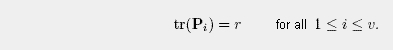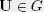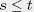Gerhard Zauner

# Quantum Designs - Definitions

A quantum design is a set D = {P1, ..., Pv} of orthogonal d x d projection matrices.

• D is said to be regular if there exists ansuch that• The degree s of D is given by the cardinality of the set• A subset of D is an orthogonal class, if its projections are pairwise orthogonal. If the sum of all its projections adds up to the identity matrix, the orthogonal class is said to be complete. A quantum design is called resolvable if it can be written as a disjoint union of complete orthogonal classes.

• A regular resolvable quantum design with degree 2 will be called an affine quantum design.

• Let G be an irreducible group of unitary d x d matrices. Then D is a quantum t-design with respect to the group G if the following equation holds for all matricesand all:For classical=commutative quantum designs (out of pairwise commuting projections) these definitions correspond to those of combinatorial design theory (the terminology is as in the book Design Theory, by T.Beth, D.Jungnickel, H.Lenz - external link).
The assignment goes via the 0/1 diagonal entries of the simultaneously diagonalized projection matrices taken as incidence functions of subsets of a set of d elements. The appropriate group G is given by the d x d permutation matrices.
Further references on (classical) combinatorial design theorie (external link).
 Diese Seite ist nur auf Englisch verfügbar